Courses

# Test: SCR Commutation Techniques

## 20 Questions MCQ Test Power Electronics | Test: SCR Commutation Techniques

Description
This mock test of Test: SCR Commutation Techniques for Electrical Engineering (EE) helps you for every Electrical Engineering (EE) entrance exam. This contains 20 Multiple Choice Questions for Electrical Engineering (EE) Test: SCR Commutation Techniques (mcq) to study with solutions a complete question bank. The solved questions answers in this Test: SCR Commutation Techniques quiz give you a good mix of easy questions and tough questions. Electrical Engineering (EE) students definitely take this Test: SCR Commutation Techniques exercise for a better result in the exam. You can find other Test: SCR Commutation Techniques extra questions, long questions & short questions for Electrical Engineering (EE) on EduRev as well by searching above.
QUESTION: 1

### The thyristor turn-off requires that the anode current

Solution:

For effective turn-off of the SCR the anode current must fall below the holding current value.

QUESTION: 2

### In case of class A type commutation or load commutation with low value of R load the

Solution:

In case of Class A commutation the requirement is that the circuit should be an under-damped RLC circuit.

QUESTION: 3

### The class A commutation or load commutation is possible in case of

Solution:

The nature of the circuit should be such that when energized from the source, current must a a natural tendency to decay to zero for load commutation to occur in a SCR circuit.

QUESTION: 4

In case of class B commutation or resonant-pulse commutation with L = 5 μH and C = 20 μC with initial voltage across the capacitor (Vs) = 230 V. Find the peak value of resonant current.

Solution:

Ip = Vs x √C/L.

QUESTION: 5

In case of class B commutation or resonant-pulse commutation with L = 5 μH and C = 20 μC with the initial voltage across the capacitor (Vs) = 230 V. Find the conduction time for auxiliary thyristor.

Solution:

Ip = Vs x √C/L
ω = 1/√LC
t = π/ω.

QUESTION: 6

An SCR is connected in series with L = 5 mH and C = 20 μF. Find the resonant frequency of the circuit.

Solution:

ω = 1/√LC.

QUESTION: 7

The type of commutation when the load is commutated by transferring its load current to another incoming thyristor is

Solution:

In the Class C type commutation also called as complementary commutation the load is commutated by transferring the current th another device.

QUESTION: 8

The type of commutation in which the pulse to turn off the SCR is obtained by separate voltage source is

Solution:

In class E commutation, another voltage source is used. It is also called as external pulse commutation.

QUESTION: 9

The natural reversal of ac supply voltage commutates the SCR in case of

Solution:

Both line and natural commuataion are used in converters.

QUESTION: 10

___________ commutation technique is commonly employed in series inverters.

Solution:

Load commuation is used in inverter in which L and C are connected in series with the load or C in parallel with the load such that overall load circuit is under damped.

QUESTION: 11

For the circuit shown in the figure below,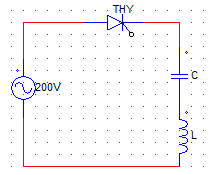C = 4 pF
L = 16 μH
Vs = 200V
The capacitor voltage after the SCR is self commutated is

Solution:

It is simply the negative of Vs as it is a series circuit & after turn off the whole voltage appears across the thyristor.

QUESTION: 12

For the circuit shown below,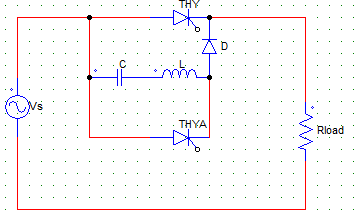The maximum value of current through thyristors T1 & TA can be given by

Solution:

I1 = (Vs/R)
T2 = (Vs√C/L).

QUESTION: 13

For the circuit shown in the figure below,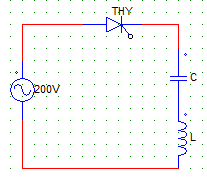C = 4 pF
L = 16 μH
Vs = 200 V
The peak thyristor current is

Solution:

The peak SCR current is Vs/2.

QUESTION: 14

For the circuit shown in the figure below, ___ type of commutation would take place for the SCR (THY1)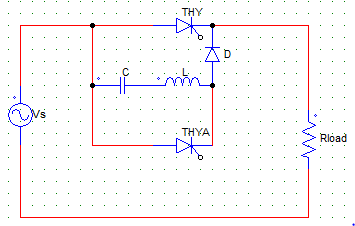Solution:

The given figure is that of a class B commutation or resonant-pulse commutation, which is a type of forced commutation technique.

QUESTION: 15

For the circuit shown in the figure below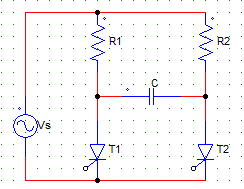R1 = 50 Ω, R2 = 100 Ω, Vs = 100 V. At the time of turn-on of the SCR, initial thyristor current is

Solution:

Io = Vs x [(2/R1)+(1/R2)].

QUESTION: 16

Natural commutation of an SCR takes place when

Solution:

Anode current (load current) becomes zero and turns off the device, hence the name line commutation.

QUESTION: 17

___________ commutation is usually used in phase-controlled rectifiers

Solution:

Line commuataion is used in converters.

QUESTION: 18

Parallel-capacitor commutation is

Solution:

Parallel capacitor is another name for forced commutation.

QUESTION: 19

Class E commutation is a/an

Solution:

As an external source is used it is a external-pulse commutation technique.

QUESTION: 20

Below is the circuit of a resonant-pulse commutation, the maximum voltage to which the capacitor C charges is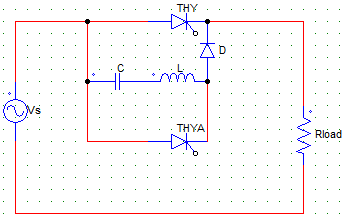Solution:

The capacitor charges from 0 to Vs with the right side plate positive.